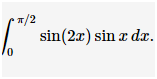## MAT 302 Assignment ProblemTrigonometric Integrals | Borough of Manhattan Community College

MAT 302  Assignment ProblemTrigonometric Integrals | Borough of Manhattan Community College

Trigonometric Integrals

Question 1

(1 point) Evaluate

sin3xcos5xdx.

Question 2

(1 point) Evaluate

tan7xsec2xdx.

Question 3

(1 point) Evaluate

tan2(6x)sec4(6x)dx.

Question 4

(1 point) Evaluate

sin7xcos3xdx.

Question 5

(1 point) Evaluate

tan3tcos3tdt.

Question 6

(1 point) Evaluate

tan2(3x)dx.

Question 7Question 8

(1 point)

Evaluate the integral

4tan2(x)dx

Question 9

(1 point)

Evaluate the integral

10sec2(x)tan(x)dx

Question 10

Evaluate the integral

2(1−tan2(x))sec2(x)dx

Question 11

(1 point) Find the indefinite integral.

sin2(x)−cos2(x)cos(x)dx

Question 12

(1 point) Find the value of π/50sin(2x)sin(x)dx∫0π/5sin(2x)sin(x)dx.

Question 13

(1 point) Evaluate

π0cos4xdx.

Question 14

(1 point) Find the indefinite integral.

cot3(x9)csc4(x9)dx

Question 15

(1 point) EvaluateQuestion 16

(1 point) Find the area of the region bounded by the graphs of the equations.

y=cos2x,y=sin(x)cos(x),x=−π2,x=π4

1. ##### Question Attachments

0 attachments —